Next: Summary Up: A Hierarchy of Geometries Previous: Defining an Affine Restriction

# From Affine to Euclidean Space

Here, by Euclidean space we actually mean space under the group of similarity transforms, i.e. we allow uniform changes of scale in addition to rigid displacements. This permits a very elegant algebraic formulation, and in any case scale can never be recovered from images, it can only be derived from prior knowledge or calibration. (You can never tell from images whether you are looking at the real world or a reduced model). In practice, Euclidean information is highly desirable as it allows us to measure angles and length ratios.

Last century, Laguerre showed that Euclidean structure is given by the location in the plane at infinity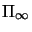of a distinguished conic, whose equation in a Euclidean coordinate system is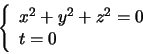(4.1)

This is known as the absolute conic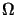[13,23]. All points lying on it have complex coordinates so it is a little difficult to picture, but for the most part it behaves just like any other conic.

Exercise 4.3   :  Show that the absolute conicis mapped onto itself under scaled Euclidean transformations. From there, show that the corresponding image conic is invariant under rigid displacements of the camera, provided that the camera's internal parameters remain unchanged.

As in the projective to affine case, prior Euclidean information is needed to recover Euclidean structure from affine space. Perhaps the easiest way to do this is to reconstruct known circles in 3D space. Algebraically, each such circle intersectsin exactly two complex points, and these always belong to.itself can be reconstructed from three such circles. Let the resulting equation be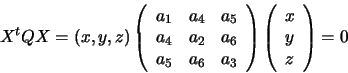A change of coordinates is needed to bringinto the form of equation (4.1). As the matrix Q is symmetric, there is an orthogonal matrix P such that: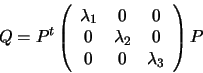Setting X'=PX, we have: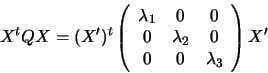With a further rescaling along each axis, we get equation (4.1).

Another way to proceed is to use the basic extended Euclidean invariant: the angle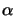between two coplanar lines L and L'. Such angles also put a constraint onand can be used to compute it. Let A and A' be the intersections of the two lines with. Let I and J be the intersections withof the (line at infinity in the) plane defined by these two lines. Laguerre's formula states that: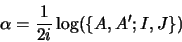We can write I=A+tA',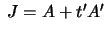. With this notation,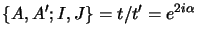. If we require that both I and J lie onwe get the constraint equations

 t2 A'TQA' + 2 t ATQA' + ATQA = 0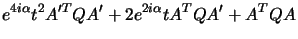= 0 (4.2)

A polynomial constraint on Q is easily derived. Eliminate t2 between the above equations: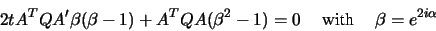extract t from this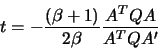and substitute into (4.2) to provide a quadratic polynomial constraint on Q: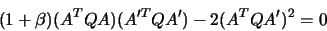Theoretically, the absolute coniccan be computed from the above constraint given 5 known angles. However, in practice there does not seem to be a closed form solution and in our experiments we have used different Euclidean constraints. But the above discussion does clearly show the relationships between the different layers of projective, affine and Euclidean reconstruction, and specifies exactly what structure needs to be obtained in each case.Next: Summary Up: A Hierarchy of Geometries Previous: Defining an Affine Restriction
Bill Triggs
1998-11-13